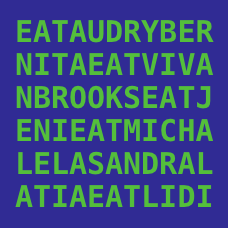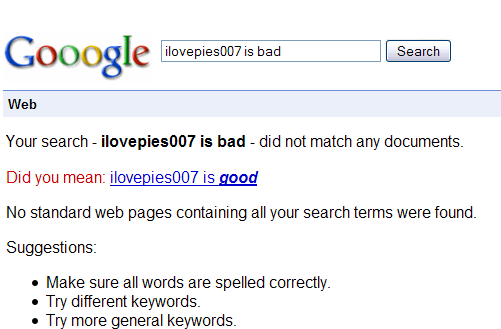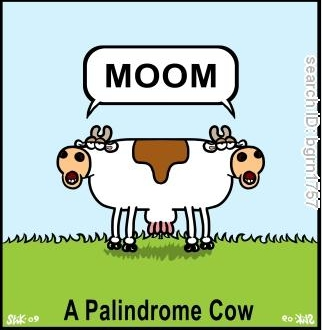Computer Science

# Strings: Level 4 ChallengesSuppose we are given two strings $a$ and $b$. We want to devise a function $f(a,b)$ that tells us how close the two strings are. A reasonable measurement would be to measure how many "edits" operations have to be made to one string in order to change it to the other. There are three possible "edit" operations:

Substitution: Replacing a single character from $a$ so that it matches $b$ costs $1$. If $a=\text{rot}$ and $b=\text{dot}$. Then $f(a,b)=1$.

Insertion: Inserting a single character also costs $1$. Ie, If $a = \text{girl}$ and $b=\text{girls}$, then $f(a,b)=1$.

Deletion: Deleting a single character also costs $1$. Ie. If $a=\text{hour}$ and $b=\text{our}$ then $f(a,b)=1$.

Given $a$ and $b$, compute $f(a,b)$.

Let the reverse of a positive integer $n$, denoted $R(n),$ be the result when the digits of the number are written backwards; for example, $R(190) = 091,$ or just $91.$

Call a positive integer $n$ brilliant if $n + R(n)$

is a multiple of 13. Let $B$ be the $10000$th brilliant number. Compute the last three digits of $B.$A palindromic-prime or PalPrime is a prime number that is also a palindrome.The first few PalPrimes are $2, 3, 5, 7, 11, 101, 131, 151, 181, 191...$. Let $S$ be the sum of the digits of the largest PalPrime $N$ such that $N<10^9$.What is the value of $S$?

×